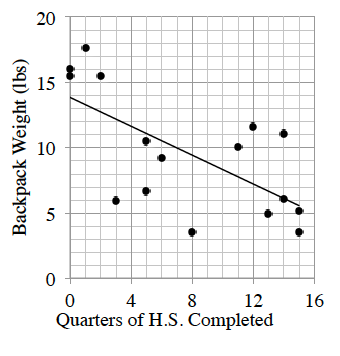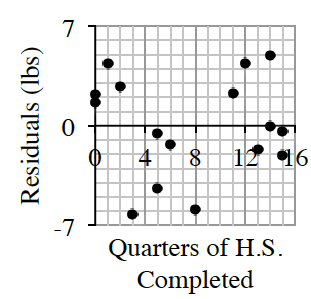### Home > CCA > Chapter 8 > Lesson 8.1.3 > Problem8-34

8-34.

Do the freshmen really have the largest backpacks, or is that just high school legend stuff? Delenn was able to weigh a random sample of student backpacks throughout the school year. She also recorded the number of quarters of high school completed by the student who owns the bag. Using spreadsheet software, Delenn found the following:

$\text{LSRL}\ y=\ 13.84-0.55x$
$r=-0.66$Qtrs Completed

Backpack Weight (lbs)

$0$

$16.03$

$0$

$15.47$

$8$

$3.52$

$12$

$11.62$

$1$

$17.66$

$5$

$6.67$

$5$

$10.48$

$13$

$4.96$

$2$

$15.47$

$6$

$9.21$

$14$

$6.10$

$14$

$11.10$

$3$

$5.96$

$11$

$10.06$

$15$

$3.54$

$15$

$5.18$1. Interpret the slope of the least squares regression line in the context of this study.

What are the units associated with the slope? Is the slope positive or negative?

On average, student backpacks get $0.55$ lbs lighter with each quarter of H.S. completed.

2. Calculate and interpret $R$-squared in context.

Square $r$ to find $R$-squared.

_____% of the variability in ____________ can be explained by a linear relationship with ____________.

3. What is the residual with the greatest magnitude and what point does it belong to?

Which point is farthest from the LSRL, or which point is farthest from the $x$-axis on the residual plot?

The largest residual is $6.2 \text{ lbs}$, relating to a student who has completed 3 quarters of H.S.

4. Using the LSRL model, estimate the weight of a backpack for a student who has completed $10$ quarters of high school. Use appropriate precision in your answer.

Let $x = 10$ in the given LSRL equation.

5. Is a linear model the best choice for predicting backpack weight in this study? Support your answer.

Does the residual plot have random scatter?